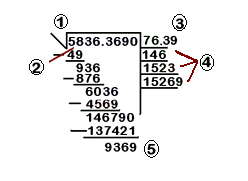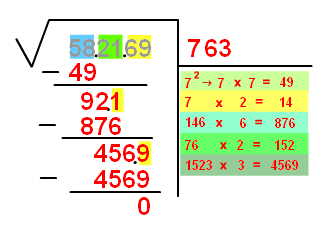# Elements of a square root and calculation step by step

## Elements of a square root

When we solve the square root with this method we have to know the parts into which the square root is divided while proceeding. The components parts are:

1. Radical, is the symbol that indicates that it is a square root.
2. Radicand, is the number which square root we are calculating.
3. Root, it is the very square root of the radicand.
4. Auxiliary lines, they will help us solve the square root.
5. Remainder, is the final number of the process to solve the square root.## Calculation of the square root step by step

When the square root of a number is calculated it is necessary to realize that the result cannot be a natural number, but a decimal number. We will study the calculation of the square roots in which the solutions are natural numbers.

To calculate the square root of a number with several decimal digits we proceed this way:

1. The number is divided into groups of two numbers, starting from the right.

2. Calculate the number that, when multiplied by himself, is closest to, but does not exceed, the first group of numbers on the left. This way, the first number of the root is obtained and we place it on the right of the radicand, on the first auxiliary line. Then the square of this number is reduced from the first group.

3. On the right side of the remainder the second group is written and the number on the right is separated.

4. The number that is on the left side of the separated number is divided by the double of the obtained root. The quotient is written on the right of the divisor and the entire part of the number that results from the division is multiplied by the same quotient. If this product can be reduced from the dividend followed by the separated number, the quotient is the number of the root; otherwise, try with a lower number.

5. Steps $$3$$ and $$4$$ are repeated until there are no more groups of radicand.

For example, let’s try it first with a number that is a perfect square:

Let's calculate the root of $$582.169$$ that is $$763$$ because $$763\cdot763=582.169$$.Step by step it would be:

1) We group in $$2$$ at a time the digits of the number $$582169$$, that is: $$58 \ \ 21 \ \ 69$$. We will write it this way:

 58.21.69

2) We look for a number that risen up to $$2$$ is close to $$58$$, in this case the number is $$7$$ since $$7\cdot7=49$$. We will write it:

 58.21.69 7 7·7=49

3) This result is reduced from $$58$$ and therefore it is: $$58-49=9$$ and we move down the two following digits, $$21$$. And we separate the last number which is $$1$$. We write it in this way:

 58.21.69 7 -49 7·7=49 92.1

4) We write the double of $$7$$ (that is to say $$14$$) in the third auxiliary line, and then the number that is on the left of the separated digit (that is $$1$$) is divided by $$14$$.

In this case $$92:14$$ and we obtain the closest integer which is $$6$$. (We write $$6$$ next to $$7$$ on the first line).

We make the subtraction, move down the next two digits and separate the last one (now $$9$$). It will be written as follows:

 58.21.69 76 -49 7·7=49 92.1 7·2=14 -876 146·6=876 456.9

5) We do the same as in the previous step. Namely, we multiply $$76$$ by two (doubling it) and, $$456$$ is divided by this double (that is, $$152$$) which gives $$3$$.

When we do the subtraction it comes to zero and, therefore, it is not necessary to continue.

We write $$3$$ in the first line, and the root we were looking for is: $$763$$.

58.21.69 763
-49 7·7=49
92.1 7·2=14
-876 146·6=876
456.9 76·2=152
-4569 1523·3=4569
0

The square root of $$582.169$$ is $$763$$.

Let's see now an example of a number that is not a perfect square, but a decimal number:

The root of $$5836,369$$

1) the number of the radicand is divided in groups of two digits. The separation is done from the decimal sign (if there is one) from right to left.

If on the side of the decimals there is not an even number of digits, it is clear that a digit will be alone: in such a case, we add a zero.

If in the integer’s side we had left a number alone, it would remain like that. In our case we must add a zero next to $$9$$. Written, this would be:

 58.36,36.90

2) We look for a number that, multiplied by itself, is $$58$$ or the closest minor number, bearing in mind that the result cannot be bigger than $$58$$.

In this case the number would be $$7$$, because $$7\cdot7=49$$. We will write:

 58.36,36.90 7 7·7=49

3) We subtract $$49$$ from the first group of two numbers of the root, which is $$9$$. We move down the following $$2$$ digits, that is $$36$$. Now we separate the last number, $$6$$. We will write:

 58.36,36.90 7 -49 7·7=49 93.6

4) We write the double of $$7$$ (that is to say $$14$$) in the third auxiliary line, and the number that is on the left side of the separated number (that was $$6$$) is divided by $$14$$. In this case the closest integer is $$6$$. (We write $$6$$ next to $$7$$ on the first line).

We subtract, move down the next two digits and separate the last one. In this case we move down $$36$$.

Since these are on the right side of the decimal point, in the first line (where we are writing) we put a decimal point as well. We will write:

 58.36,36.90 76, -49 7·7=49 93.6 7·2=14 -876 146·6=876 603.6

5) Now, we repeat the previous step. We multiply $$76$$ by two (we double it) and $$603$$ is divided by this double (that is $$152$$) which gives $$3,9$$; therefore, taking the entire part, we have $$3$$.

The operation to be done is $$1523\cdot3$$. We write the result under the last rest that we had and do the subtraction.

We move down the following two digits. We write 3 in the first line. This is written as:

 58.36,36.90 76,3 -49 7·7=49 93.6 7·2=14 -876 146·6=876 603.6 76·2=152 -4569 1523·3=4569 1467.90

6) The same previous step is repeated. We multiply the root that we have written by now $$(76,3)$$ by $$2$$ but ignoring the decimal point, that is to say $$763\cdot2=1526$$.

The result is written on the following auxiliary line, and the first four numbers of the remainder $$(1467)$$ are divided by the result of the multiplication, giving the number $$9$$ as the first number that is not a zero of the division.

We write $$9$$ in the line of the root and $$9$$ is multiplied by $$15269$$, that is $$137421$$. This is the number that we have to subtract from the last remainder that was $$146790$$ which is $$9369$$.

 58.36,36.90 76,3 -49 7·7=49 93.6 7·2=14 -876 146·6=876 603.6 76·2=152 -4569 1523·3=4569 1467.90 763·2=1526 -137421 15269·9=137421 9369

At this point, we can say that approximately the square root of $$5836,369$$ is $$76,39$$ with a reminder of $$9369$$.

This operation, used as an example, is not finished. If we continue it would give $$76,391321$$. The process is repeated until the reminder is zero. But we observe that the square root is not an integer, since $$5836,369$$ is not a perfect square.